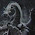## Sunday, June 23, 2019

### Somehow I Received an A in Both 2nd Semester and 3rd Semester Algebra

It sure is not showing now!  Please identify where I went wrong.  I am trying to write gCode to excavate a half-circle in a block of aluminum.  (Really a half cylinder.)  I will advance x by 0.5" with each pass.  (It is a .5" diameter mill.)  What is the formula for z?

Circle is:
(x-h)2 = (a-k)2 = r2
where r is radius, h,k is center of circle. Solve for z, since x is known. To simplify this I am calculating upward motion, which I will go -z for vertical position.
x2 - 2xh + k2 + z2 - 2zk +k2 = r2
-2xh +h2 + z2 -2zk +k2 = r2 - x2
h2+ z2 - 2zk + h2 = r2 - x2 + 4xh + k2
z2 - 2zx = r2 - x2 + 4xh + k2
z = r2 _x2 + 4xh = k2 + 2k
But plugging in r=4.5, x=0, h=4.5, k=4.5 (the left side of the workpiece) produces an obvious error.

1.2.